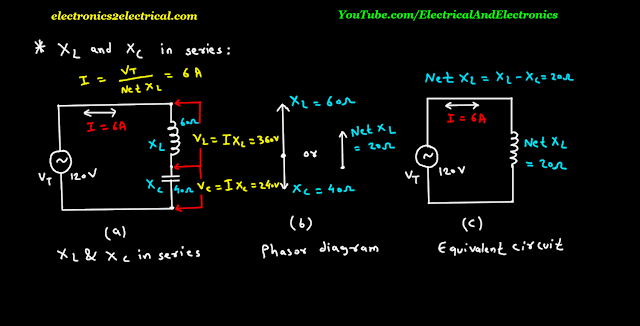X L and X C in Series

0 like 0 dislike

Inductive reactance X L and Capacitive reactance X C in series.

Inductive reactance X L of value 60 ohm and Capacitive reactance X C of value 40 ohm are connected in series.

120 volts A C voltage is applied.

The current I is same in both the inductive reactance and capacitive reactance.

The inductive reactance and capacitive reactance has opposite phase angles.

So inductive reactance and capacitive reactance in series the net reactance is the difference between X L and X C.

Which results in less reactance than either one.

The figure c shows the equivalent circuit with net reactance 20 ohm and A C voltage source of 120 volts.

Since the net reactance is inductive because the value of inductive reactance is more as compare to capacitive reactance.

Due to net inductive reactance the current lags the applied voltage by 90 degree.

The voltage drop across X L in figure A is V L is equal to Current I into X L which is equal to 6 ampere into 60 ohm which is 360 volts.

The voltage drop across X C in figure A is V C is equal to Current I into X C which is equal to 6 ampere into 40 ohm which is 240 volts.

The individual voltage drop is more than applied voltage but the sum of series voltage drop is 120 volts.

The Voltage V L and V C are opposite.

The voltage across inductive reactance leads series current by 90 degree.

The voltage across capacitive reactance lags the series current by 90 degree.

The voltage across inductive reactance V L and voltage across capacitive reactance X C are 180 degree out of phase.

And they are of opposite polarity.

Now suppose the values of inductive reactance X L is replaced with inductive capacitance X C and value of X C replaced with value of X L.

So X C will be 60 ohm and X L will be 40 Ohm in this case the net reactance would be 20 ohm X C.

The current will be 6 ampere.

This time current will lead the voltage by 90 degree.